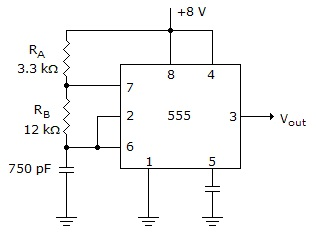# Digital Electronics - Multivibrators and 555 Timer

### Exercise :: Multivibrators and 555 Timer - General Questions

11.

What is the difference between an astable multivibrator and a monostable multivibrator?

 A. The astable is free running. B. The astable needs to be clocked. C. The monostable is free running. D. none of the above

Explanation:

No answer description available for this question. Let us discuss.

12.

Is there any limit to the number of times that a 74123 can be retriggered?

 A. yes B. no

Explanation:

No answer description available for this question. Let us discuss.

13.

Determine tHI and tLO for the circuit given below.A. tHI = 7.95s, tLO = 6.24s B. tHI = 6.24s, tLO = 7.95s C. tHI = 3.97s, tLO = 3.21s D. tHI = 3.21s, tLO = 3.97s

Explanation:

No answer description available for this question. Let us discuss.

14.

The output of the astable circuit ________.

 A. constantly switches between two states B. is LOW until a trigger is received C. is HIGH until a trigger is received D. floats until triggered

Explanation:

No answer description available for this question. Let us discuss.

15.

If a diode is connected across resistor RB (positive end up) in the given figure, what is the new duty cycle of the output waveform?A. 56% B. 44% C. 21.6% D. 17.4%